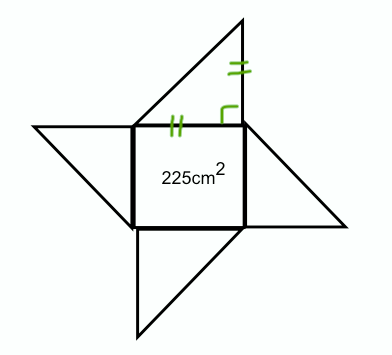### Pythagoras

Thursday, February 26, 2009
Vocabulary:
Hypotenuse - The hypot
enuse is on the opposite of the right angle and is the longer side of the triangle.
Legs - Are the shorter sides of the triangle.
R.A.T.
- Right Angle Triangle.

Theor
em - A theorem is a demonstrated statement that has been proved or needs to be proven. Pythagoras' theorem was a² + b² = c² .
Greek - A native or citizen of Greece. and that's it for the vocabulary.

Pythagoras was a Greek who lived 2500 years ago. He was an "uber" smart mathematician. He is the one who came up with the theory of a² + b² = c². Pythagoras is important because his mathematical theories and formulas are still used today.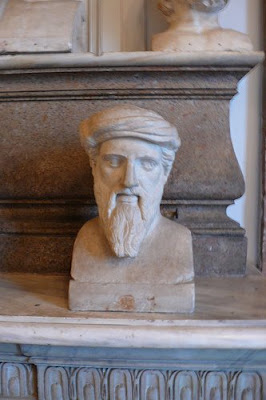This shape is a right angle triangle (R.A.T.). The legs are the shorter side of the triangle (a and b). Across the right angle, is the hypotenuse. The hypotenuse is always the longest side. The hypotenuse is c. The formula for a right angle triangle is a² + b² = c². a can either be the vertical line, or the bottom line. The same goes to b.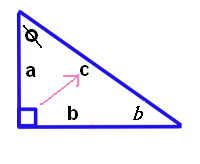This shape is a square and it has four right angles. The lines on the square just help you see that its equal on all sides. All together the square equals 360 degrees and to get that all you do is time 90 degrees by 4 which is 360.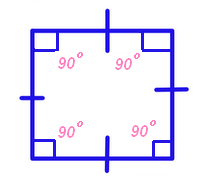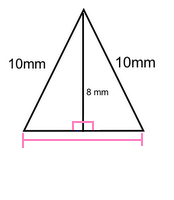You need to find out how long each side of the square is. Then that will give you side 'a' and 'b' for the triangles since they're both the same length. So then find the length of the hypotenuse, which is 'c'. After you find that out you can now label the triangle and add up all the sides to find the perimeter.